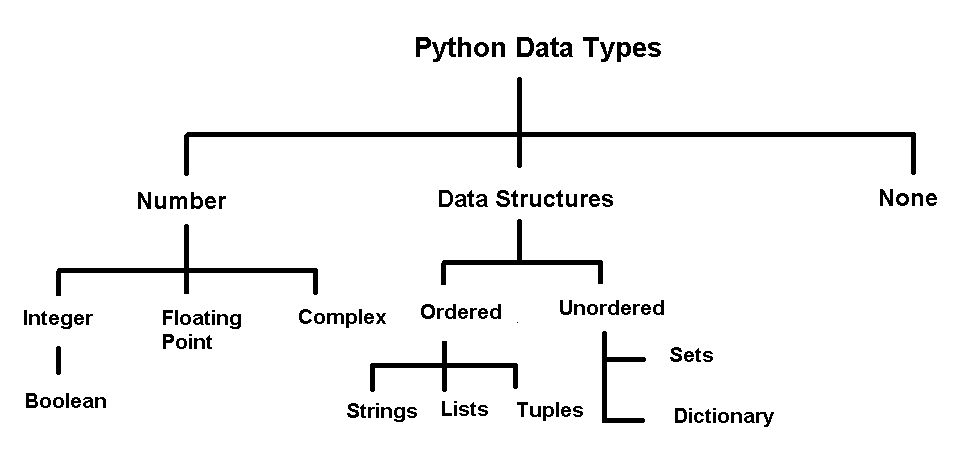# Python Data Types ExamplesData types are fundamental to programming languages and they define the type of values get stored in a Python variable. Here you will practice python data types which are similar to other programming languages, yet python don’t have strict type checking like C++, Java, etc. Strict type checking means the you need to declare the type of data before storing value in a variable. For example, int Num; Here ‘int’ means integer type.It is recommended that you try these problems on your own and then compare the results. May be you may come up with a better way to solve the problem.

### Program 1: Write a program that store number data types , output the type and the data.

#### Solution:

``````# Filename : Number_type.py
# write a program that store number types, output the values and type

# number types are - integer, float , complex , and boolean

integer_type = 23
float_type = 344.66
complex_type = 3 + 2j
bool_type1 = True
bool_type2 = False

# Print values and their types

print(integer_type)
print(type(integer_type))

print(float_type)
print(type(float_type))

print(complex_type)
print(type(complex_type))

print(bool_type1)
print(type(bool_type1))

print(bool_type2)
print(type(bool_type2))``````

#### Output:

``````23

344.66

(3+2j)

True

False
``````

### Program 2: Writing a program to store ordered data types such as strings, lists, and tuples and display their value. Also, output their type information.

#### Solution:

``````# Filename : ordered_data_type.py
# Writing a program to display values of ordered data type with type information

# python ordered types are string, list, and tuple

myString = "Himalayas"

myList = ["Milk","Banana", "Bread", "Chocolate", 400]

myTuple = (100, "Notesformsc", "ComputerScience")

# print the values and the type

print(myString)
print(type(myString))

print(myList)
print(type(myList))

print(myTuple)
print(type(myTuple))``````

#### Output:

``````Himalayas

(100, 'Notesformsc', 'ComputerScience')
``````

### Program 3: Write a program to display third letter of the string “MOUNTAIN”.

#### Solution:

``````# Filename : Display_letter.py
# Writing a program to display third letter of the string "MOUNTAIN"

myString = "MOUNTAIN"

# index start at 0; therefore, third letter is index is 2

myLetter = myString

# print the output

print("Third letter is",myLetter)``````

#### Output:

``Third letter is U``

The post Python Data Types Examples appeared first on Notesformsc.

This post first appeared on Notesformsc, please read the originial post: here